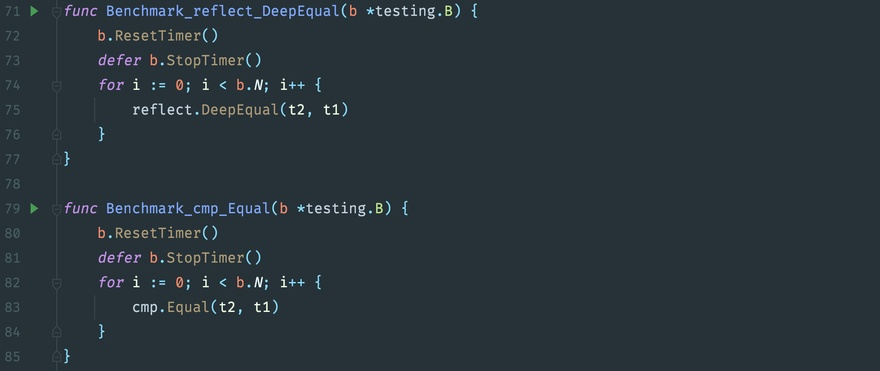You can use `reflect.DeepEqual` and `cmp.Equal` to compare two structs of the same type to see if they are equal, or you can use hard-coded comparisons, see below how they perform.Define a `struct` with multiple data types, e.g.

 ``````1 2 3 4 5 6 `````` ``````type T struct { X int Y string Z []int M map[string]string } ``````

## reflect.DeepEqual

This is a built-in function

 ``````1 `````` ``````reflect.DeepEqual(t2, t1) ``````

## cmp.Equal

Import library `github.com/google/go-cmp/cmp`

 ``````1 `````` ``````cmp.Equal(t2, t1) ``````

## Hard-coded comparisons

For a particular kind of struct comparison

 `````` 1 2 3 4 5 6 7 8 9 10 11 12 13 14 15 16 17 18 19 20 21 22 23 24 25 26 27 `````` ``````func customEqual(t1, t2 T) bool { if t1.X != t2.X { return false } if t1.Y != t2.Y { return false } if len(t1.Z) != len(t2.Z) { return false } for i, v := range t1.Z { if t2.Z[i] != v { return false } } if len(t1.M) != len(t2.M) { return false } for k := range t1.M { if t2.M[k] != t1.M[k] { return false } } return true } ``````

## Test Results

 `````` 1 2 3 4 5 6 7 8 9 10 `````` ``````goos: darwin goarch: amd64 cpu: Intel(R) Core(TM) i7-4870HQ CPU @ 2.50GHz Benchmark_reflect_DeepEqual Benchmark_reflect_DeepEqual-8 688875 1595 ns/op 483 B/op 18 allocs/op Benchmark_cmp_Equal Benchmark_cmp_Equal-8 139269 8421 ns/op 2840 B/op 52 allocs/op Benchmark_custom_Equal Benchmark_custom_Equal-8 10542481 111.9 ns/op 0 B/op 0 allocs/op PASS ``````

In terms of implementation time, it takes more time.

 ``````1 `````` ``````cmp.Equal > reflect.DeepEqual * 5 > customEqual * 75 ``````

If the program only compares fixed structs for equality, consider writing your own comparison function for performance.